Search IntMath
Close

450+ Math Lessons written by Math Professors and Teachers

5 Million+ Students Helped Each Year

1200+ Articles Written by Math Educators and Enthusiasts

Simplifying and Teaching Math for Over 23 Years

# How to find the equation of a quintic polynomial from its graph

By Murray Bourne, 26 Mar 2016

My earlier article on How to find the equation of a quadratic function from its graph has generated a lot of interest and many visits.

"How could we go about figuring out the equation of other types of graphs?

Say I have the following quintic graph. How would I figure out the function?"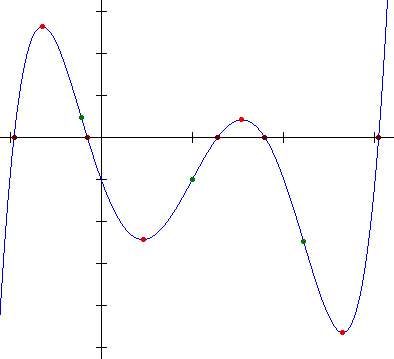The graph has several key points marked:

1. There are 5 x-intercepts (black dots)
2. There are 2 local maxima and 2 local minima (red dots)
3. There are 3 points of inflection (green dots)

[For some background on what these terms mean, see Curve Sketching Using Differentiation].

That should be plenty for us to find a good approximation for the equation of the function.

I'm going to assume it's a quintic (that is, a polynomial function of degree 5), not only because Mike said it was, but also the curve gets very steep at the far left and far right, suggesting it's a quintic. Of course, it is possible it has other curves beyond the domain shown, but we can only work with what we've been given.

## General form of a quintic

A polynomial function of degree 5 (a quintic) has the general form:

y = px5 + qx4 + rx3 + sx2 + tx + u

We'll find the easiest value first, the constant u.

## Finding the constant

From the graph we see that when x = 0, y = −1. Substituting these values in our quintic gives u = −1

So our quintic becomes:

y = px5 + qx4 + rx3 + sx2 + tx − 1

## Finding the x-intercepts

Our aim is to set up a system of 5 equations with 5 unknonws.

Next, we'll substitute in the x-intercepts (where y = 0). It's too hard to estimate the places where the curve cuts the x-axis, so we construct a grid, as follows: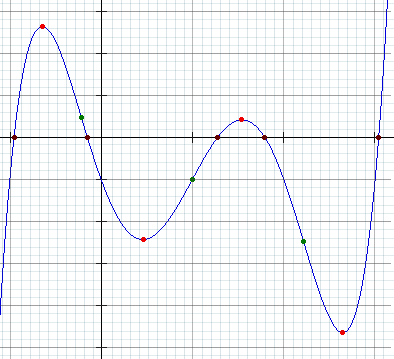Using this grid, we can estimate where the black dots are on the x-axis:

x = −0.96, −0.15, 1.28, 1.8, 3.05

We substitute those 5 points one at a time into our general quintic equation and obtain the following 5 equations (Of course, I'm using software to do these steps):

− 0.8154p + 0.8493q − 0.8847r + 0.9216s − 0.96t − 1 = 0 ... 

− 0.00008p + 0.0005q − 0.003r + 0.0225s − 0.15t − 1 = 0 ... 

3.4360p + 2.6844q + 2.0972r +1.6384s + 1.28t − 1 = 0 ... 

18.8957p + 10.4976q + 5.832r + 3.24s + 1.8t − 1 = 0 ... 

263.9363p + 86 5365q + 28.3726r + 9.3025s + 3.05t − 1 = 0 ... 

Next, we solve those 5 simultaneous equations (using a computer algebra system) and obtain:

p = 0.9882235373

q = −4.960882158

r = 4.978373717

s = 5.015096102

t = −6.043658926

This means our quintic equation is approximately:

y = 0.988x54.96x4 + 4.978x3 + 5.015x2 − 6.043x − 1

(Of course, I've used full available accuracy throughout, but have rounded my answers for clarity.)

## Is the equation correct?

In the earlier article about finding the equation of a quadratic curve, we learned that there are an infinite number of possibilities if we only use the x-intercepts.

However, how good is the solution we just found?

We'll check it graphically, then analytically.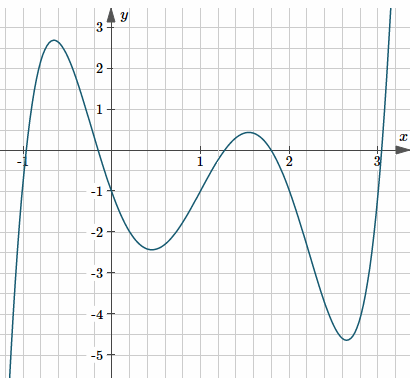The above derived graph is certainly close to the original curve given.

Now let's analytically examine if the other key points on the graph make sense.

## Local maxima and minima

The local maxima and local minima (the "tops of the hills" and "bottoms of the valleys") are found by finding the first derivative, setting it equal to 0, then solving for x.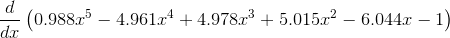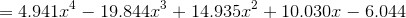This equals 0 when x = −0.648, 0.458, 1.551 or 2.655.

Substituting these back into the equation for the quintic gives us the two local maxima:

(−0.648, 2.680) and (1.551, 0.427),

and the two local minima:

(0.458, −2.436) and (2.655, −4.653).

A check on the graph above shows these are very close to the red dots on the curve.

## Points of inflection

There are three points of infleciton shown on the graph. Let's find the points of inflection using the quintic equation I found.

We find the second derivative and set it equal to zero.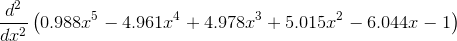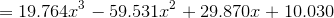This second derivative equals zero when x = −0.226, 1.004, or 2.234.

Substituting these back into the equation for the quintic gives the points of inflection:

(−0.226, 0.552), (1.004, −1.005) and (2.234, −2.540).

These are very close to the green dots on the curve.

So we can be confident we have a very good approximation for the equation of the quintic.

## The original equation

The astute amongst you would have noticed the equation I obtained is actually very close to the following equation with integer values for the coefficients:

y = x55x4 + 5x3 + 5x2 − 6x − 1

In fact, this was the original curve, as given in the page from where the graph came.

### 7 Comments on “How to find the equation of a quintic polynomial from its graph”

1. Rene Grothmann says:

You could just multiply over (x-v[k])

>v&:=[-0.96, -0.15, 1.28, 1.8, 3.05]
[-0.96, -0.15, 1.28, 1.8, 3.05]
>function p(x) &= product(x-float(v[k]),k,1,5)

(x - 3.05) (x - 1.8) (x - 1.28) (x + 0.15) (x + 0.96)

>&expand(-p(x)/p(0))

5 4
0.9882235377453956 x - 4.960882159481885 x
3 2
+ 4.978373716099981 x + 5.015096102762597 x - 6.043658925318761 x
- 1.0

2. Theophile Habiyambere says:

Great! Thanks a lot for the explanations provided here. It is really giving us a clear picture on how to find equations given their curves.

3. Murray says:

@Rene: Thanks for the alternate method!

4. SF says:

Thanks for this post!
Really explains
how to find the equation, manually.

Q1: What is the software tool
you say was used for this calculation?

Q2: in Rene Grothmann's comment:
looks like program code...what is the programming language used?
How can we try out ourselves?

Q3: Any online calculator
to (easily) input the available points
and obtain the final equation as a result?

(not being lazy...just trying to see if there's an existing automated solution
to make it easy! 🙂

Thanks!
SF

5. SF says:

can you supply a list of the 10 points
in (x , y) format?
ie:
x y
1 ,10
2, 25
etc...

Would make it easier to test out
other tools and compare results

Thanks again, Murray! 🙂
SF

6. Murray says:

@SF: (1) You can use Wolfram|Alpha for solving simultaneous equations. This example shows how: https://www.wolframalpha.com/input/?i=x%2By%2Bz%3D10,+x-y-3z%3D4,+x-5y-3z%3D40

(2) Rene's code looks like Matlab.

(3) You can always use MS Excel to construct a polynomial through your given points, like I did in the earlier article: https://www.intmath.com/blog/mathematics/how-to-find-the-equation-of-a-quadratic-function-from-its-graph-6070

Regards
Murray

7. Murray says:

@SF: The easiest way to do that would be to set it up in Excel and use the actual equation I give right at the end. But you would need to include negative values for x as well - not a good idea to start at x = 1.

### Comment Preview

HTML: You can use simple tags like <b>, <a href="...">, etc.

To enter math, you can can either:

1. Use simple calculator-like input in the following format (surround your math in backticks, or qq on tablet or phone):
a^2 = sqrt(b^2 + c^2)
(See more on ASCIIMath syntax); or
2. Use simple LaTeX in the following format. Surround your math with $$ and $$.
$$\int g dx = \sqrt{\frac{a}{b}}$$
(This is standard simple LaTeX.)

NOTE: You can mix both types of math entry in your comment.